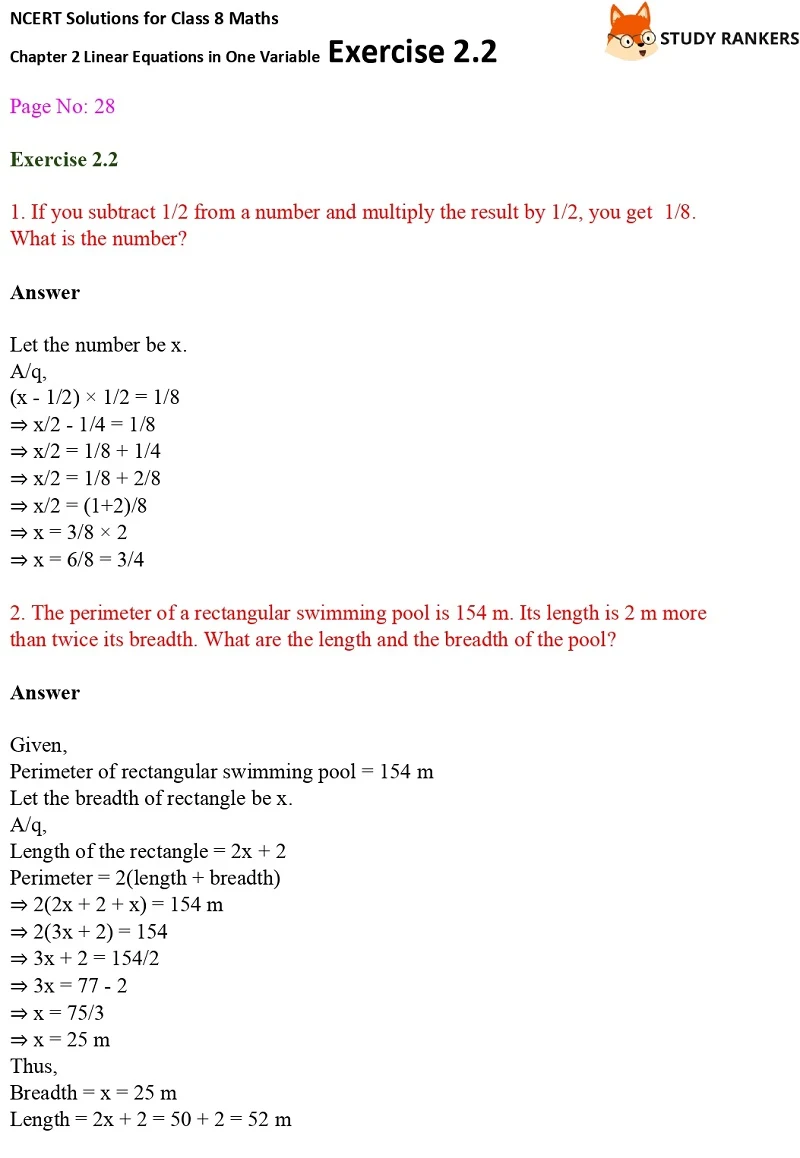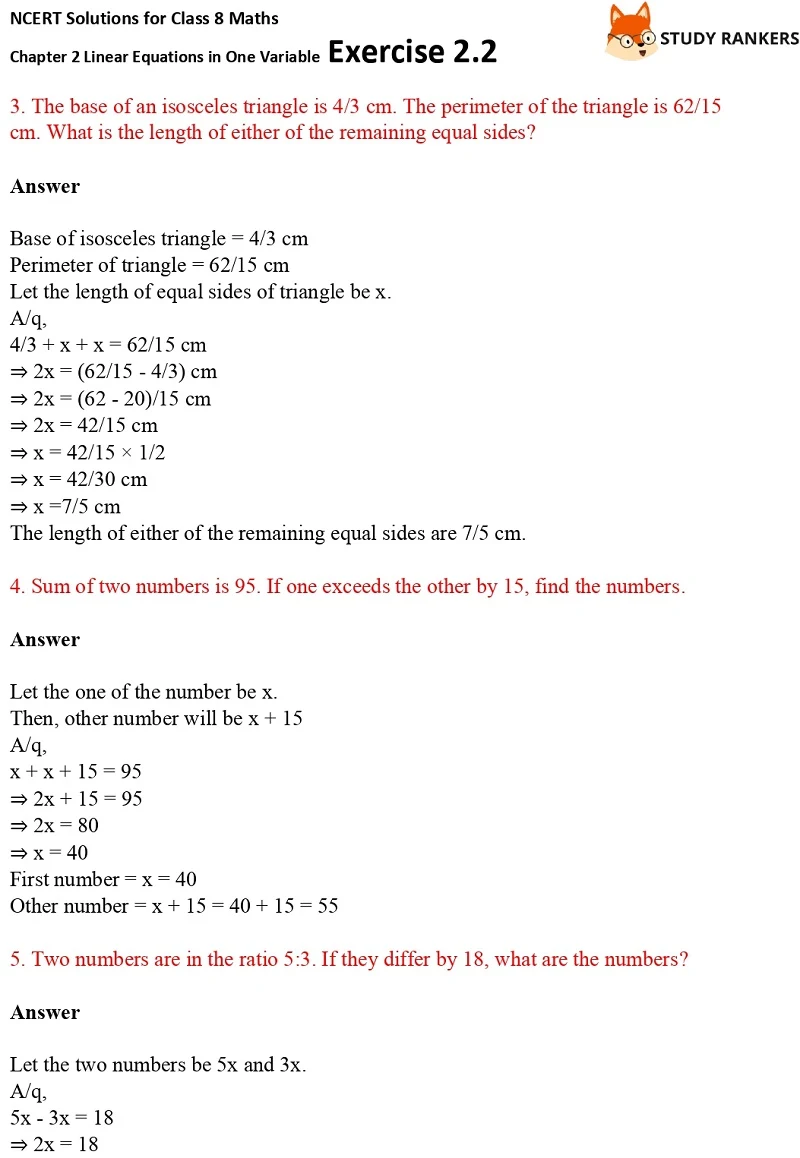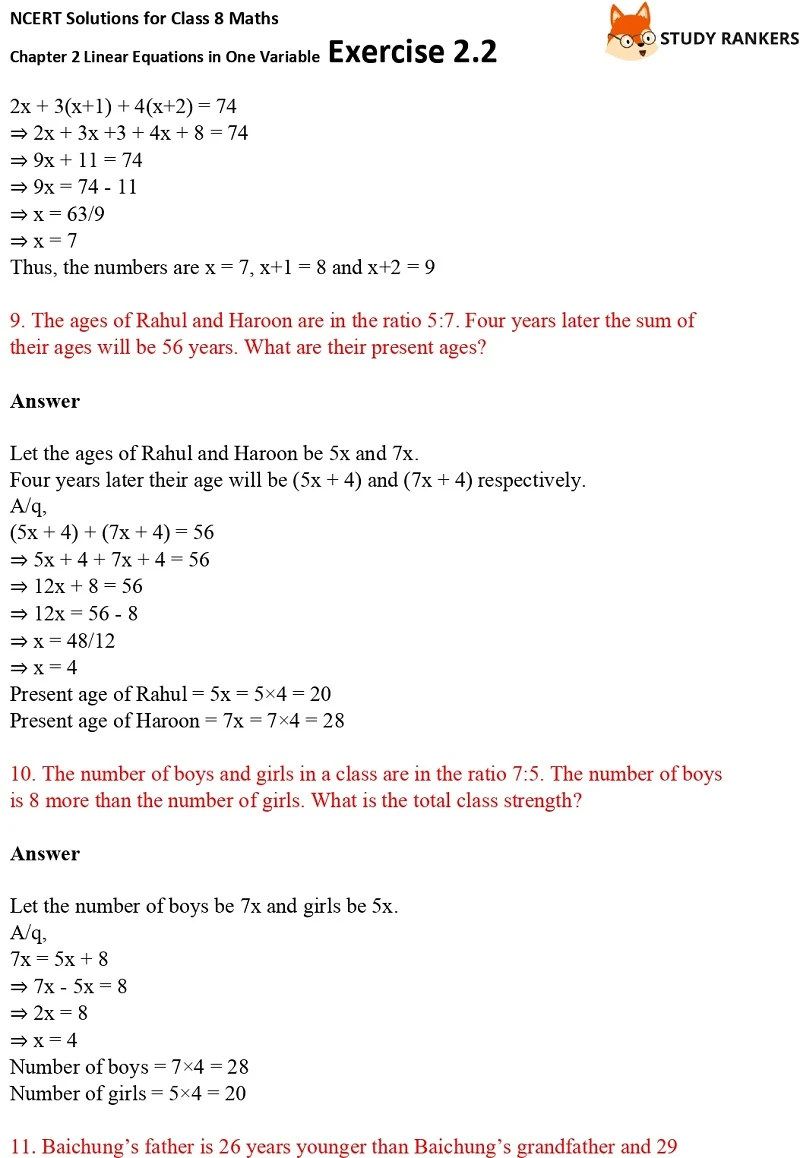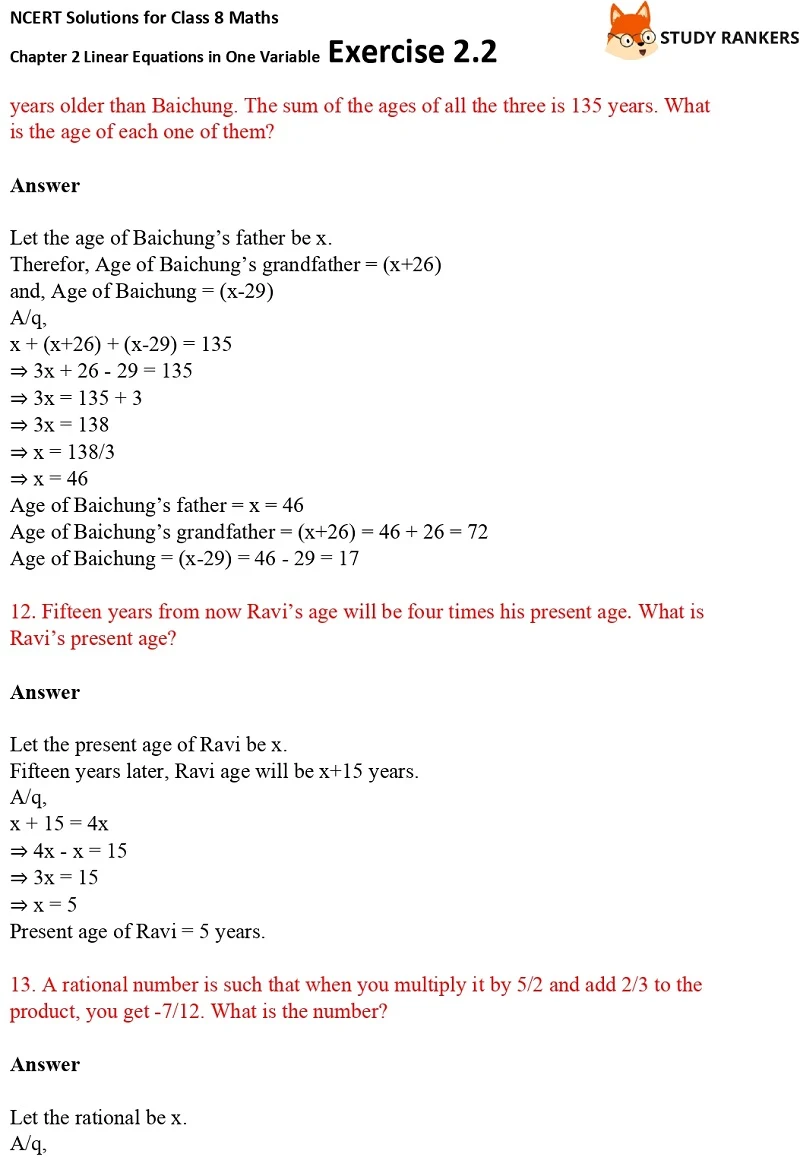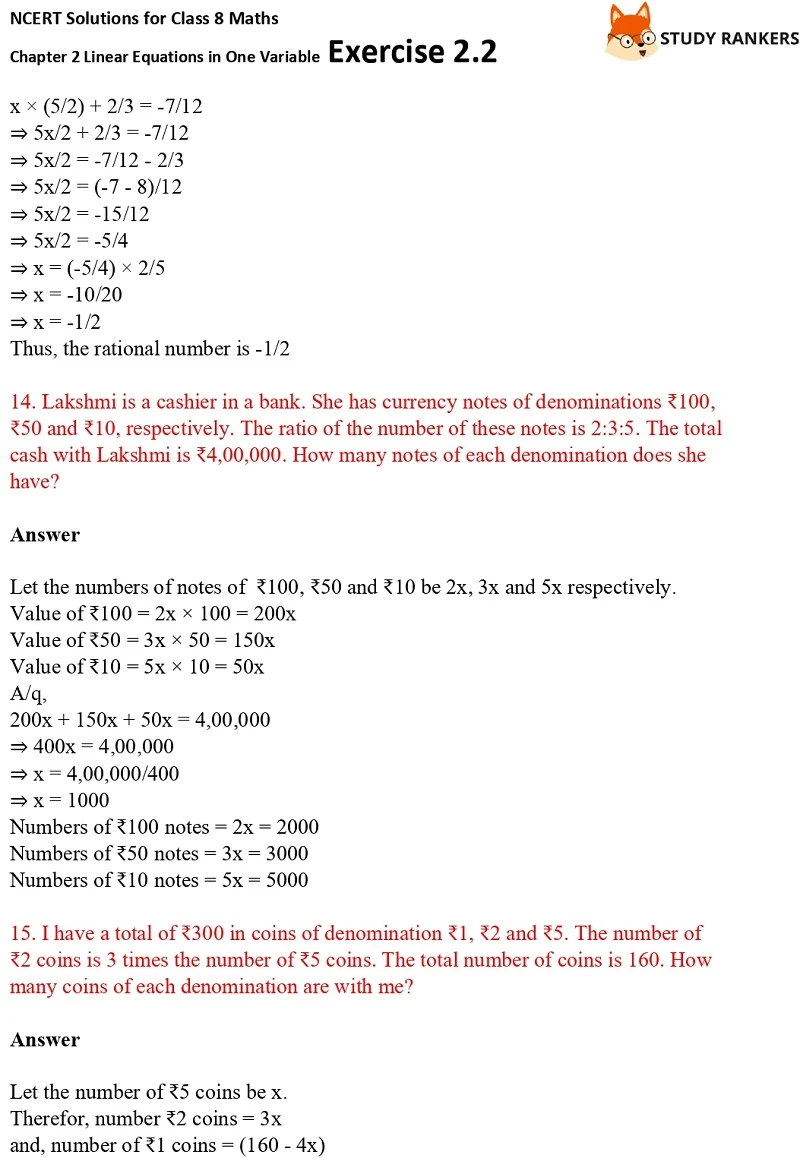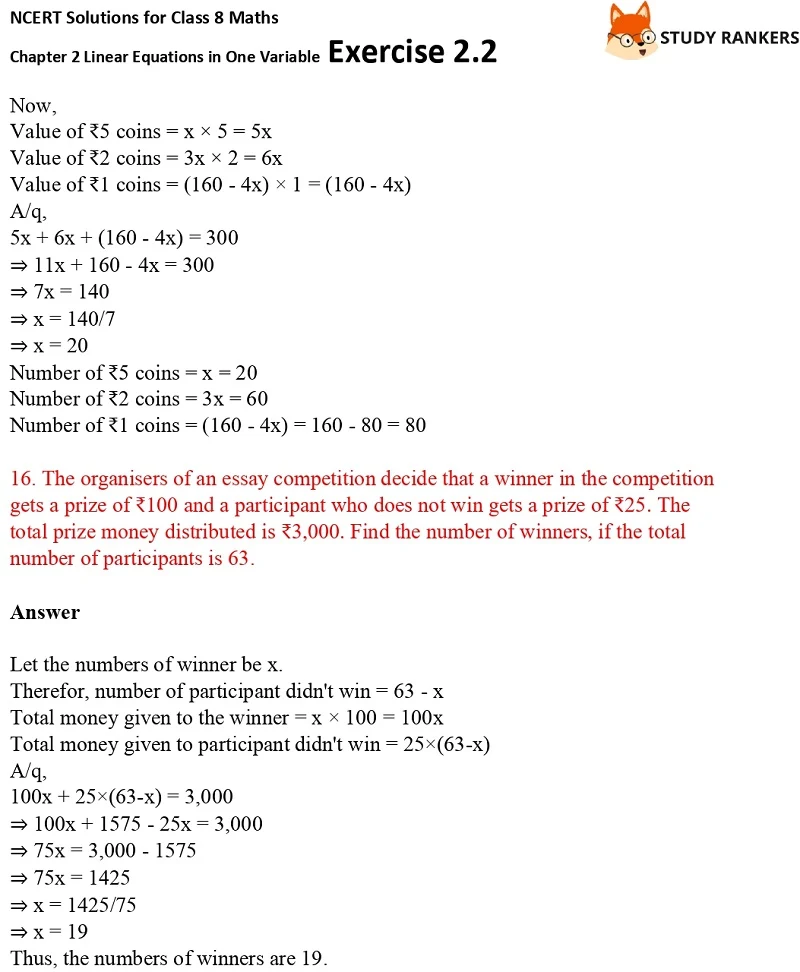# NCERT Solutions for Class 8 Maths Ch 2 Linear Equations in One Variable Exercise 2.2

NCERT Solutions for Class 8 Maths Ch 2 Linear Equations in One Variable Exercise 2.2 are provided which are helpful in solving difficult problems given in the exercise. Exercise 2.2 Class 8 Maths NCERT Solutions can be sometimes really tough so students can take help from these. Through the help of these NCERT Solutions you can complete homework on time.

You will find NCERT Solutions for Class 8 Maths which are accurate that will help you out in obtaining higher grades. In the exercise 2.2, you will find word problems that you need to solve by putting them into equations.